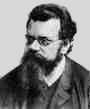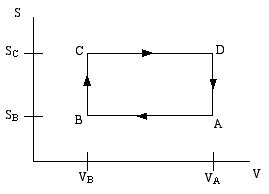Home

PHY 418: Statistical Mechanics I
Prof. S. Teitel stte@pas.rochester.edu ---- Spring 2012

## Problem Set 2

Due Wednesday, February 22, in lecture

• Problem 1 [10 points total]

A thermodynamic engine can be thought of as a cyclic process by which heat (TdS) pumped from a "hot" reservoir into a system (the engine) is converted into mechanical work (pdV) done by the engine. The most famous such example is the Carnot cycle. Another such cycle is the Otto cycle, schematically shown in the diagram below as a directed path in the volume-entropy plane. The Otto cycle is a rough approximation to the operation of a gasoline engine.The gas is compessed adiabatically (constant entropy) in the step A to B. Then it is heated isochorically (constant volume) in the step B to C (this step corresponds to the combustion of the gas in the gas engine). The gas is then expanded adiabatically in step C to D (this is the power stroke). Finally the gas is cooled isochorically to bring it back to its initial state; this is step D to A.

Step B to C extracts heat QBC from the "hot" reservoir to heat the gas, while step D to A returns heat QDA = − QAD to the "cold" reservoir (usually the ambient atmosphere). The difference is the work done by the engine.

If the engine efficiency is defined as ratio of the net heat converted to work, divided by the heat withdran from the hot reservoir

ε = (QBC − QAD)/QBC

then assuming the gas is an ideal gas, show that the efficiency of the Otto cycle is given by,

ε = 1 − (VB/VA)(Cp − CV)/CV

(Hint: for this problem, read the supplemental notes to lecture 6.)

• Problem 2 [10 points total]

Consider a box of volume V. The box is split exactly in half by a thermally conducting imovable wall. Equal quantities of the same type of ideal gas fill each half of the box, and the system is in equilibrium. The total energy of the gas is fixed at ET.

a) Show that the pressure of the gas on each side is the same (remember, the wall is imovable, so you can't just appeal to mechanical equilibrium).

b) Using the formular derived in lecture for the number of states Ω(E) of an ideal gas at total energy E, find the most likely value for the energy of the gas on one side of the box.

c) Using Ω(E), if P(E) is the probability distribution for the gas on one side of the box to have energy E, show that the relative width (i.e. the width divided by the average) of P(E) is proportional to 1/N1/2, for sufficiently large N, where N is the number of particles in the gas. This shows that the fluctuation of E away from its average becomes negligibly small in the thermodynamic limit of N going to infinity. (For "width" you may use half width at half height or any other reasonable definition.)

• Problem 3 [20 points total]

Consider a system of N distinguishable non-interacting objects, each of which can be in one of two possible states, "up" and "down", with energies +ε and −ε. Assume that N is large.

(a) Working in the microcanonical ensemble, find the entropy of the system S(E, N) as a function of fixed total energy E and number N (Hint: it is useful to consider the numbers N+ and N of up and down objects).

(b) Using your result from (a) find the temperature T as a function of energy E and number N. Show that T will be negative if E>0.

(c) What happens if such a system (1) with T1<0 comes into thermal contact with another such system (2) with T2>0? Does T1 increase or decrease? Does T2 increase or decrease? In which direction does the heat flow?

• Problem 4 [10 points total]

Consider the same situation as in problem 3, i.e. a system of N distinguishable non-interacting objects, each of which can be in one of two possible states, "up" and "down", with energies +ε and -ε. Assume that N is large.

(a) Working in the canonical ensemble, find the Helmholtz free energy A(T, N) as a function of temperature T and number N.

[Note: Having found Ω(E,N) in problem 3, you could compute the canoncial QN(T) by taking the Laplace transform of Ω(E,N) with respect to E. Don't do it this way! Instead, compute QN(T) by directly summing the Boltzmann factor over all states in the phase space.

(b) Starting from A(T, N) of part (a), find the canonical entropy and express it as a function of the average energy E and number N. Show that your result agrees with your answer for the entropy in the microcanonical ensemble, as computed in problem 3, in the large N limit.